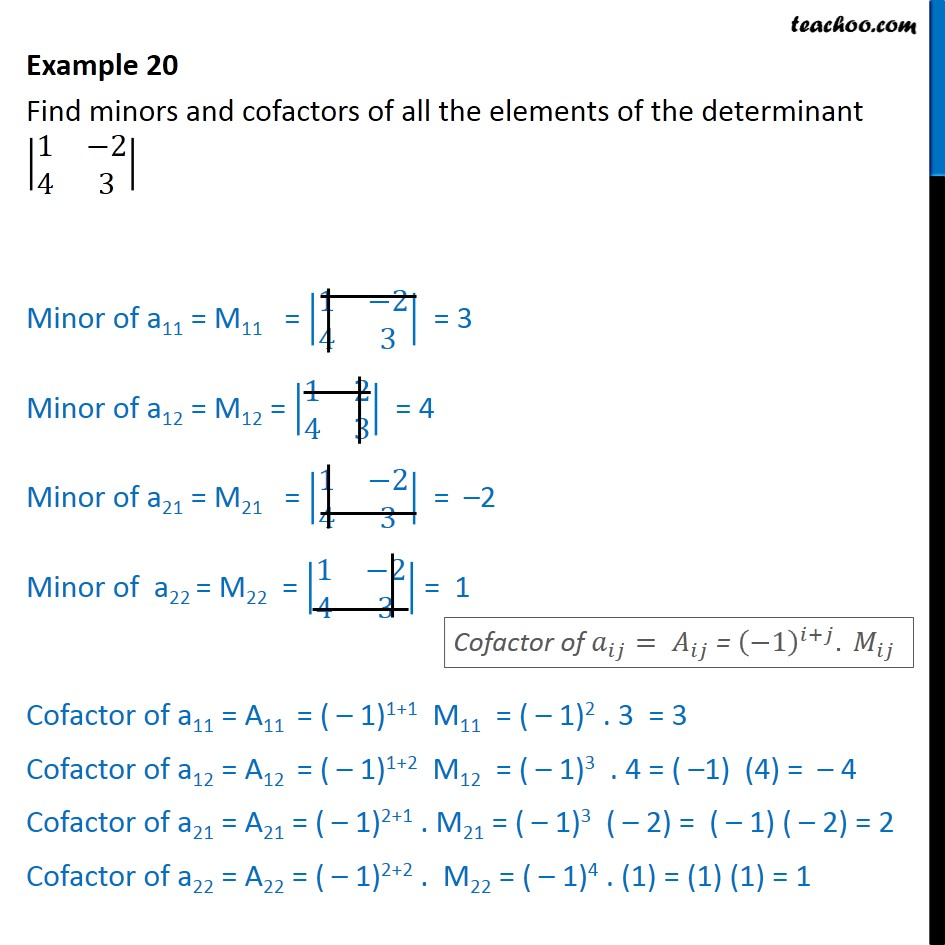Finding Minors and cofactors

Chapter 4 Class 12 Determinants
Concept wiseLearn in your speed, with individual attention - Teachoo Maths 1-on-1 Class

### Transcript

Example 9 Find minors and cofactors of all the elements of the determinant 1 2 4 3 Minor of a11 = M11 = 1 2 4 3 = 3 Minor of a12 = M12 = 1 2 4 3 = 4 Minor of a21 = M21 = 1 2 4 3 = 2 Minor of a22 = M22 = 1 2 4 3 = 1 Cofactor of a11 = A11 = ( 1)1+1 M11 = ( 1)2 . 3 = 3 Cofactor of a12 = A12 = ( 1)1+2 M12 = ( 1)3 . 4 = ( 1) (4) = 4 Cofactor of a21 = A21 = ( 1)2+1 . M21 = ( 1)3 ( 2) = ( 1) ( 2) = 2 Cofactor of a22 = A22 = ( 1)2+2 . M22 = ( 1)4 . (1) = (1) (1) = 1